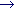# Case Study: Poisson Equation

• Poisson1 (source):
problem:
• find approximative solution of the Poisson equation• on a rectangular grid for given given sources f and boundary values
remarks:
• grid approximates continuum, solution by Jacobi iteration:• source example: "electronic lens"
• implementation uses two alternating U matrices (avoids additional copies)
• there are much better algorithms (SOR, multigrid)!
• Poisson2 (source):
parallelisation:
• PARALLEL around it loop, DO around sweep loop
synchronisation:
• barriers between sweeps are necessary
• automatic by END DO
data locality:
• variables in sweep (incl. sweep itself) private by default
• it private, undefined after END PARALLEL
(LASTPRIVATE only possible with DO)
solution: print in SINGLE block inside parallel block
• error: private (multiple writes)
problem: each thread computes only partial error
•error has different value in each thread
•loop exit condition doesn't work, deadlock possible (try n=20)
• Poisson3 (source):
global exit criterion necessary
summation of error to (shared) total_error
• can't use REDUCTION at PARALLEL, since summation is done only at the end of the PARALLEL block.
• can't use REDUCTION at DO in sweep, since the return value is - like all local variables - thread private.
initialisation of total_error in SINGLE
summation inside CRITICAL:
• only one thread at a time inside critical block
• threads run through it serially
• must be short (performance problem)
BARRIER before test of total_error
• Poisson4 (source):
idea: sweep returns total error, main program is simple
problem: all variables in sweep are local
solution: variable in extra module (or COMMON block) are shared
summation of partial errors as before, but hidden in sweepPeter Junglas 16.2.1999